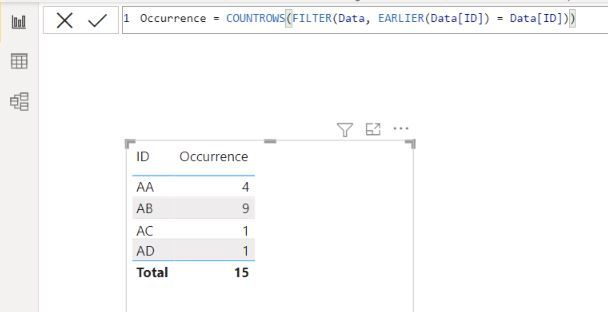cancel
Showing results for
Did you mean:Frequent Visitor

## Calculated column to get number of occurrences is returning values that squares the correct count

Hello.. I have been searching all day for an answer to my dilemma.  I wanted to get the count of how many times the value in a column appears in the column. I have found lots of solutions involving COUNTROW(FILTER formula that also involves EARLIER function. I am amazed that it has worked for a lot of the users who needed that solution.  But for some reason, it does not work for me.  The resulting value squares the correct count.

To illustrate, for example I have below data.  Table name is "Data".  I want column B to return the count of occurrence of the ID in column A:So I made this formula in Power BI Desktop via a calculated column.  As you can see, the result is squaring the actual count:I hope someone can help me with this.  Any possible reason why this is happening? And any solution for this?

1 ACCEPTED SOLUTIONResolver I

It seems the implicit aggregation for the value was left on Sum.

Here are two approaches to address the issue with a sample file.

Regards

2 REPLIES 2Resolver I

It seems the implicit aggregation for the value was left on Sum.

Here are two approaches to address the issue with a sample file.

RegardsSuper User

@raellaylo , Create a measure

countrows(filter(allselected(Table), Table[ID] =max(Table[ID]))

I think simple measure

countrows(Table)

should also work